## SQL Queries in Pandas

##### Python Data Science: Learn Python SQL Queries in Panda in Detail with Real Examples
Written by |28-Apr-2019 | 0 Comments | 320 Views

As we have stated previously in data science that pandas can load many types of files in which SQL database file is one of those. Pandas can not only load the SQL file but also can run the SQL queries to extract the data. In this section, we will see how we can perform differently from SQL queries in pandas.

This portion is also related to the query language introduction which we will be learning in later lectures.

So, let’s start this. Figure 1 Source:

And the ER diagram which shows the column names of the database is as follows: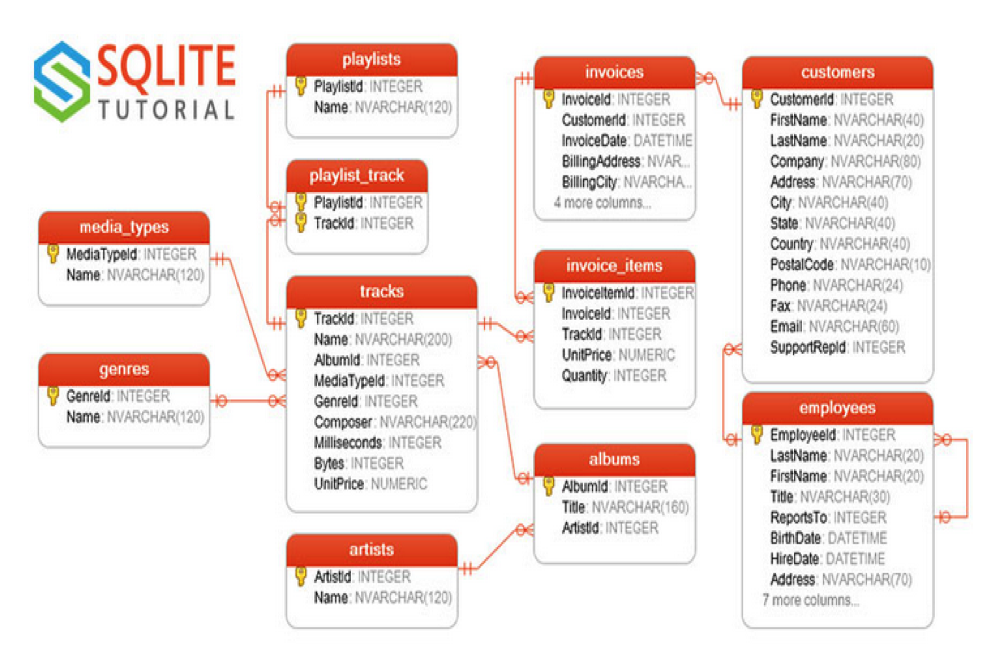Figure 1

We can see there is a table of customers, playlists, employees, etc. So now we will access this database with the help of python pandas.

How to connect to Database:

To connect to the database first, we have to import the module of sqlite3 in python by using pip command. After importing, we will use the connect method, and in the parameter, we will give the path SQLite DB file. It is to be noted that sqlite3 only works for local SQL database for other databases like Postgres we have to use psycopg2 to connect the database. After connecting to the database, we then use the object of connection as a parameter in creating the data frame of pandas. The practical implementation of establishing a connection is as follows:

`import pandas as pd`
`import sqlite3`
`conn = sqlite3.connect('/home/lalatoofani/Desktop/DataScience/chinook.db')`
`df = pd.read_sql("Select * from customers limit 5",conn)`
`df`

As discussed above we first import the modules that made the connection and ran a query to get the first five rows of the data and the second parameter is the object of the connection.

Conditional Queries:

Just like we have made conditions in normal pandas data frames, we can also make conditional queries in SQL. These conditional queries include like, where, and, etc.

We will see all the types one by one:

First, we will see where clause. Following is the code to implement the where clause:

`import pandas as pd`
`import sqlite3`
`conn = sqlite3.connect('/home/lalatoofani/Desktop/DataScience/chinook.db')`
`df = pd.read_sql("Select * from customers where Country='USA'",conn)`
`df`

The output will be as follows: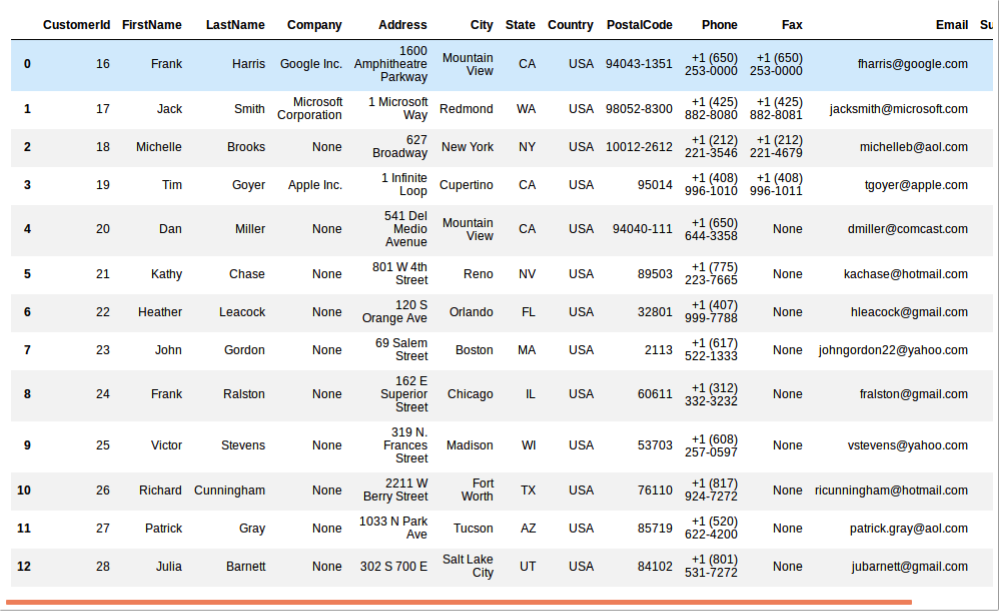Figure 2

Similarly, we can apply other operations too.

But there is some limitation that we can apply this SQL query at just starting the or while creating the data frame after that when a data frame is created, and we will use normal ways of pandas to handle the data.

Reset Index:

When we drop the row, the index values got misplaced which can sometimes cause the loop not work well for that we first reset the index. By resetting the index, the values at the index column get arranged from 0 to the n number of rows which make the pandas to do looping better. Following is the example of resetting the index:

`import pandas as pd`
`df = pd.read_csv('titanic.csv')`
`df = df.dropna(axis=0)`
`df`

The output that is generated after dropping the columns that contain null value is as follows: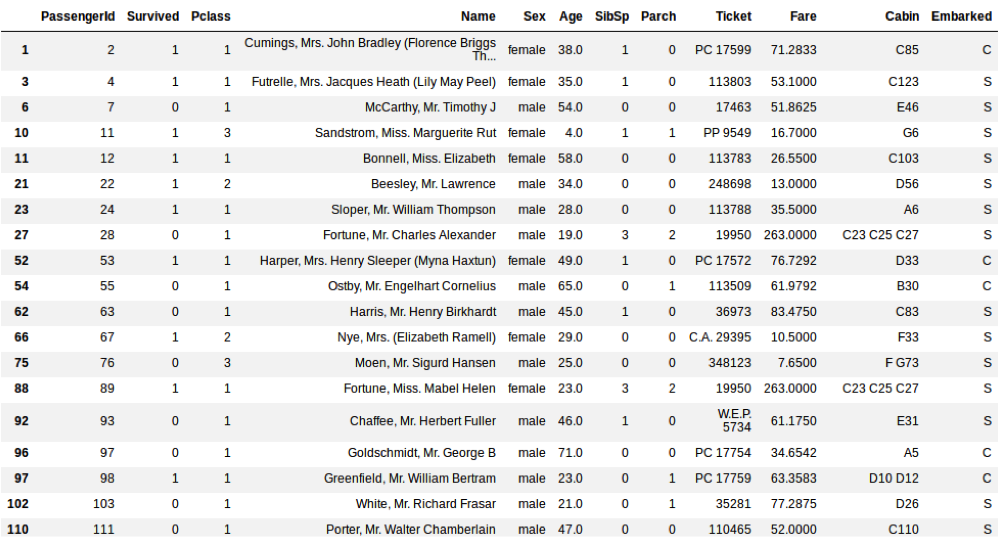Figure 3

As we can see that the index values are not aligned there 1, 3,6 10….. which will make confusion at the time of looping so we will reset index as follows:

`df = df.reset_index().drop(['index'],axis=1)`
`df`

By executing this line of code, the index will be reset and starts from the value 0 as shown in figure 4: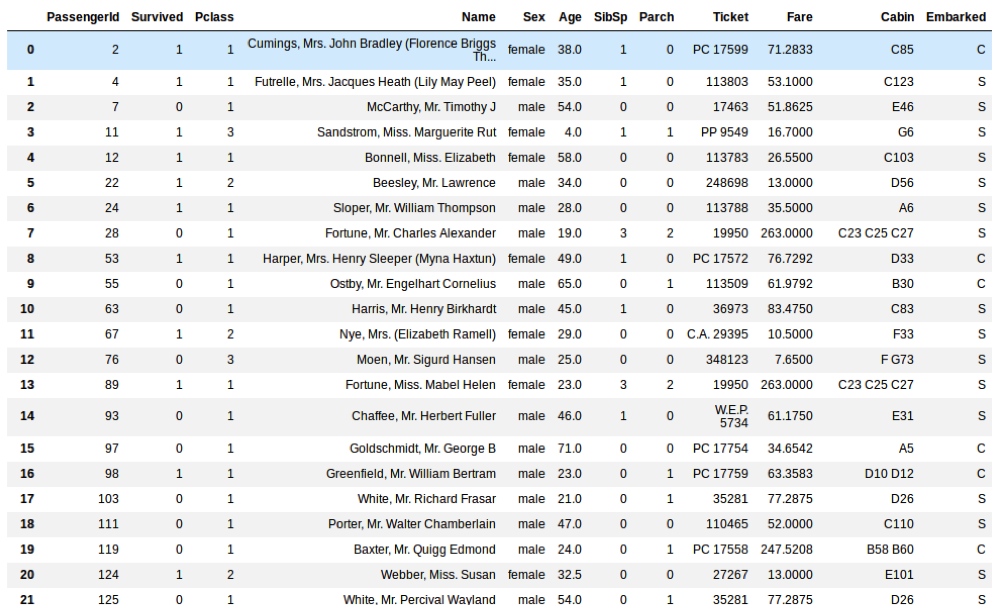Figure 4

We drop the index column because after resetting the panda's data frame retain the older version index so the index will be drop so that no additional column is generated in our data frame that may cause an obstruction in future.

Data Visualization using Pandas:

We can also plot the data using pandas. Pandas use matplotlib functionality to generate the graphs. By making the column name along with the plot function will plot the data. Now we will see how we can plot the data.

We will first make a data frame of the Titanic dataset and then rename the male as 0 and female as 1 and then we will what is the frequency of causalities of male vs. female. Following is the code for implementation:

`import pandas as pd`
`df = pd.read_csv('titanic.csv')`
`df['Sex'] = df['Sex'].apply(lambda x:0 if x=='male' else 1)`

`df = df[df['Survived']==1]`
`df['Sex'].plot()`

The output is as follows: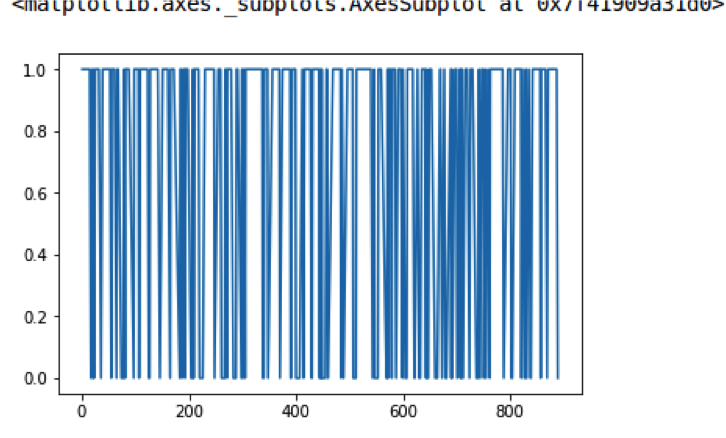Figure 5

The blue line indicates female and white gap indicates male so we can deduct from the above figure that more women survived the deadly incident as compared to men.

Concatenation of Data frames:

Some time we have to merge two data frames for such purpose concat function is used. Concat function merger the two data frames which have the same headers. Now the question of why it is used? It is extensively used when we do not load the whole data at once rather than load the data in parts. Sometimes the computer memory is not sufficient to load the big file of data which sometimes leads to crash the computer or took so much time. For that reason, we use some parallel computing technique to load the data chunk by chunk and then concatenate the data into the previous one. Following is the code to implement the concatenation of two data frames:

`Import pandas as pd`

`df1 = pd.DataFrame({'A': ['AA0', 'AA1', 'AA2', 'AA3'],`
`                    'B': ['BB0', 'BB1', 'BB2', 'BB3'],`
`                    'C': ['CC0', 'CC1', 'CC2', 'CC3'],`
`                    'D': ['DD0', 'DD1', 'DD2', 'DD3']},`
`                     index=[0, 1, 2, 3])`
`df2 = pd.DataFrame({'A': ['AA4', 'AA5', 'AA6', 'AA7'],`
`                    'B': ['BB4', 'BB5', 'BB6', 'BB7'],`
`                     'C': ['CC4', 'CC5', 'CC6', 'CC7'],`
`                     'D': ['DD4', 'DD5', 'DD6', 'DD7']},`
`                     index=[4, 5, 6, 7])`
`df3 = pd.DataFrame({'A': ['AA8', 'AA9', 'AA10', 'AA11'],`
`                    'B': ['BB8', 'BB9', 'BB10', 'BB11'],`
`                     'C': ['CC8', 'CC9', 'CC10', 'CC11'],`
`                     'D': ['DD8', 'DD9', 'DD10', 'DD11']},`
`                     index=[8, 9, 10, 11])`

`df = pd.concat([df1,df2,df3])`

The output of the above program is as follows: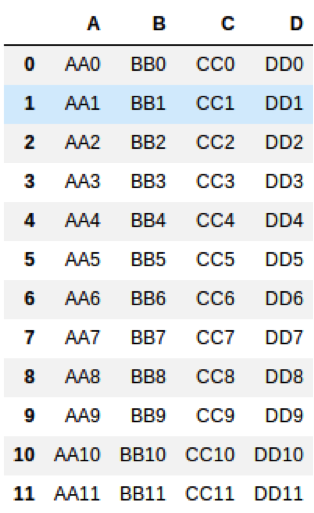Figure 6

This is all about the pandas. These basics will be used when we will be doing machine learning and in image processing too but the majority portion will be used while doing machine learning. So before entering into the machine learning make your hand dirty on pandas. In the next section we will be creating a small project in pandas for analyzing the shortest distance.